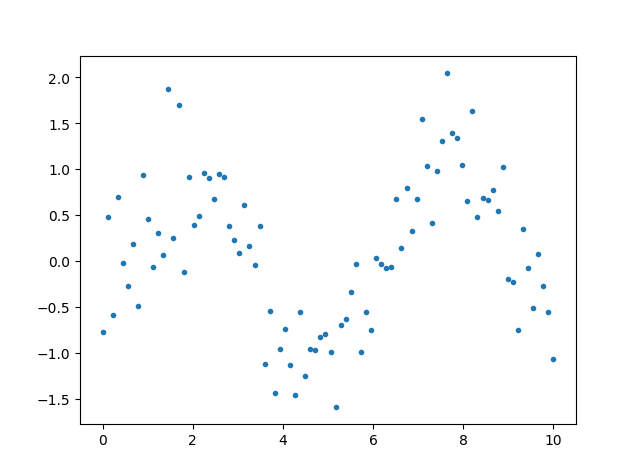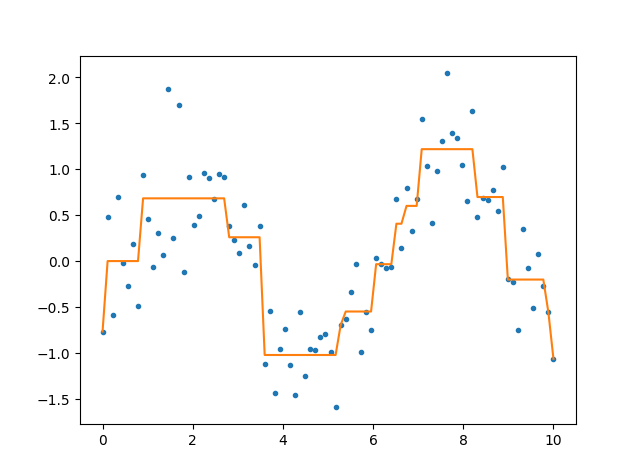## 【Decision Tree】理论与实现

2017年05月22日    Author:Guofei

## 简介

• 每个节点两个走向
• 每个节点1个变量
• 变量可以重复使用

• 易于理解和解释
例如，收集专家的历史数据，用决策树可以总结出他的经验。
• 和其它模型相比，数据的预处理往往是不必要的。例如，不需要归一化 ，也 不用填充空值
• 可以同时处理分类变量和连续变量。其它模型很多要求数据类型单一
• 白盒模型，可以轻松推出逻辑表达式
• 计算量少，大量数据可也以快速得出良好结果
• 有变量筛选功能
• 决策树可很好地扩展到大型数据库中，同时它的大小独立于数据库的大小。

• 样本各类别数量不同时，结果更偏向于类别多的。 （除了朴素贝叶斯之外的大多数模型都存在这个问题）
• 过拟合
• 忽略自变量之间的相关性（因为每个节点只能选取1个feature）

• 有监督
• 既可以做分类，也可以做回归
• 也可以用于变量筛选

## 理论推导

Quinlan在1986年提出ID3,1993年提出C4.5
Breiman在1984年提出CART算法

### 定义

• internal node表示一个特征或属性
• leaf node 表示一个类

### 输入数据

$x_i=(x_i^{(1)},x_i^{(2)},…,x_i^{(n)})$是一个样本,有n个维度
$y_i \in { 1,2,…K }$类标记
N是样本容量

### 模型2

lost function是正则化极大似然函数

### ID3(算法)

ID3递归算法：
step1：对于当前要计算的节点，找到信息益增最大的Feature，记为$A_g$
step2：如果信息益增小于$\epsilon$，生成节点，把实例数最大的类作为这个节点的类，递归结束
step3：否则，用$A_g$中的每一个可能值$\alpha_i$构建下多个子节点，递归转到每一个子节点。

### C4.5

C4.5是ID3的改进，在ID3的基础上增加一个步骤：剪枝。

#### part1，用信息增益率生成树

信息增益率来选择属性,而不是信息益增

step1：对于当前要计算的节点，找到信息益增率最大的Feature，记为$A_g$
step2：如果信息益率增小于$\epsilon$，生成节点，把实例数最大的类作为这个节点的类，节点变成叶节点，递归结束
step3：否则，用$A_g$中的每一个可能值$\alpha_i$构建下多个子节点，递归转到每一个子节点。

#### part2，剪枝(pruning)

$C_\alpha(T)=\sum\limits_{t=1}^{\mid T \mid} N_t H_t(T)+\alpha \mid T \mid$
where,
$\mid T\mid$是叶节点个数

$\alpha$控制模型复杂度

1. 扫描叶节点，$T_A$是修剪后的叶节点，如果$C_\alpha(T_A) \leq C_\alpha(T)$，那么剪枝
2. $T=T_A$,继续上一步，直到所有节点都不再能修剪为止

C4.5的特点：

1. 能够完成对连续属性的离散化处理；
2. 能够对不完整数据进行处理。
3. 其缺点是：在构造树的过程中，需要对数据集进行多次的顺序扫描和排序，因而导致算法的低效。
4. C4.5只适合于能够驻留于内存的数据集，当训练集大得无法在内存容纳时程序无法运行。
5. 生成是局部选择，剪枝是全局选择

### CART

• 二叉树，左支代表是，右支代表否
• 既可以用于分类，也可以用于回归

#### 最小二乘回归树生成算法

$$R_1(j,s)=\{ x \mid x^{j} \leq s \}$$
$$R_2(j,s)=\{ x \mid x^{j} > s \}$$

$$\min\limits_{j,s}\bigg[\min\limits_{c_1}\sum\limits_{x_1 \in R_1(j,s)}(y_i-c_1)^2+\min\limits_{c_2}\sum\limits_{x_2 \in R_2(j,s)}(y_i-c_2)^2\bigg]$$

#### CART生成算法

$Gini(X)=\sum_{k=1}^K p_k(1-p_k)$

特征A下，集合D的gini index定义为：
$Gini(D,A)=\dfrac{\mid D_1 \mid}{\mid D\mid}Gini(D_1)+\dfrac{\mid D_2 \mid}{\mid D\mid }Gini(D_2)$（与conditonal entropy相似的概念）
where,
$D_1,D_2$是被$A=\alpha$分割后的集合

step1：对于当前要计算的节点，找到gini index最小的Feature和划分$A=\alpha$
step2：如果样本数量小于阈值，或者gini index小于阈值$\epsilon$，生成节点，把实例数最大的类作为这个节点的类，节点变为叶节点，递归结束
step3：否则，构建子节点，递归转到每一个子节点。

#### CART剪枝算法

$C_\alpha(T)=C(T)+\alpha\mid T\mid$
step1: 改变$\alpha$，例如,排序的$0,\alpha_1,\alpha_2,…,+\infty$可以得到一组子树:$$\{ T_0,T_1,...T_n \}$$。

step2: 用交叉比较的方法找到$T_0,T_1,…T_n$中最优的。

## python实现：

### 环境准备

sklearn的一个缺点：pruning not currently supported。只实现了pre-pruning

1. 安装graphviz
conda install graphviz

2. 安装软件graphviz，官网：

### 代码

#### step1：做出模型

from sklearn import datasets

from sklearn import tree
clf=tree.DecisionTreeClassifier()
clf=clf.fit(dataset.data,dataset.target)


#### step2：把规则输出

tree.export_graphviz(clf,out_file="tree.doc"  )#输出到doc


#### step3：规则可视化

import graphviz
import os
os.environ["PATH"] += os.pathsep + 'C:/Program Files (x86)/Graphviz2.38/bin/'
dot_data = tree.export_graphviz(clf, out_file=None,feature_names=dataset.feature_names,  class_names=dataset.target_names,filled=True, rounded=True,special_characters=True) #参数是配置颜色等
graph = graphviz.Source(dot_data)
graph.view('hehe.pdf')
graph.save('abc.pdf')
graph #在jupyter中有用clf.predict(train_data)#判断数据属于哪个类别
clf.predict_proba(train_data)#判断属于各个类别的概率
clf.feature_importances_#变量重要性指标，各个属性的gini系数归一化后的值
#例如，print(pd.DataFrame(list(zip(data.columns,clf.feature_importances_))))


## 用CART拟合的例子

import numpy as np
from sklearn.tree import DecisionTreeRegressor

x=np.linspace(0,10,90)
x.shape=-1,1
y=np.sin(x)+np.random.normal(size=x.shape)*0.5clf=DecisionTreeRegressor(max_depth=4)
clf.fit(x,y)
ynew=clf.predict(x)

import matplotlib.pyplot as plt
plt.plot(x,y,'.')

plt.show()

plt.plot(x,y,'.')
plt.plot(x,ynew)
plt.show()## 后注（备用）

### 可视化输入方法（备用）

1. 安装pydotplus：
https://github.com/carlos-jenkins/pydotplus
2. 安装graphviz
conda install graphviz

3. 安装软件graphviz，官网：

4. 加入环境变量：
import os
os.environ["PATH"] += os.pathsep + 'C:/Program Files (x86)/Graphviz2.38/bin/'


import pydotplus
import os
os.environ["PATH"] += os.pathsep + 'C:/Program Files (x86)/Graphviz2.38/bin/'
dot_data = tree.export_graphviz(clf, out_file=None)
graph = pydotplus.graph_from_dot_data(dot_data)
graph.write_pdf("iris.pdf")


from IPython.display import Image
dot_data = tree.export_graphviz(clf, out_file=None,feature_names=iris.feature_names,  class_names=iris.target_names,filled=True, rounded=True,special_characters=True)
graph = pydotplus.graph_from_dot_data(dot_data)
Image(graph.create_png())


## 参考文献